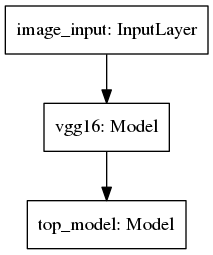I had some trouble retraining models provided with Keras as there were some changes in the API. The basics of building classification models through bottlenecking and retraining dense layers of already trained models (such as VGG16) is well described in Francois Chollet blog post about this topic and this post aims only at providing corrections so to actually run the code with Keras 2 functional API. This post is more a discussion of what needs to be done than step by step tutorial.You may also run into similar problems when trying to use any of models provided at Keras model repository as they are no longer Sequential models but just Models, and this format is currently less documented. Corrections are easy though.

New functional API makes it easy to make some crazy connections between layers as all of them are accessible through layers’ Tensors. Moreover, each layer can take a tensor as an argument.

Let’s say that you just want to run a prediction on a batch of images using VGG16. You cannot run

``````model = VGG16(include_top=True, weights='imagenet')
...
model.predict_generator(image_generator, number_of_images // batch_size)

``````

As this will give you an error stating that the input size is wrong – if you look inside, VGG16 is a function and you need to give it a tensor with size (image_width,image_height,3) or (3,image_width,image_height). Because we are giving it a batch of images our input tensor input_shape is a 4-touple (batch_size,image_widht…). However using the functional API we can provide our own input layer and connect it to a input tensor of VGG16 model.

``````model = VGG16(include_top=True, weights='imagenet')

inputs = Input(shape=(img_width,img_height,3),name = 'image_input')
prediction = model(inputs)

whole_model=Model(inputs=inputs,outputs=prediction)
``````

We can use the whole_model Model to batch predict without a problem

In case of using VGG16 to create a bottleneck features (as in Francois Chollet’s blog) the batch generation of the features and training the fully-connected classifier can be done as explained in the blog. The tricky part is to stick those two together. We not only need to connect VGG16 model to an input layer but also connect the VGG16 output tensor to our dense model. Using Functional API I declared the top model through:

``````first= Input(shape=(4, 4, 512))
second=Flatten(name="flatten")(first)
top_model= Model(inputs=first, outputs=predict)
top_model.compile(optimizer='rmsprop',
loss='binary_crossentropy',
metrics=['accuracy'])
``````

And found the weights through:

``````early_stopping = EarlyStopping(monitor='val_loss', patience=10, verbose=True, mode='auto')

filepath="bottleneck_fc_model2.h5"
checkpoint = ModelCheckpoint(filepath, monitor='val_acc', verbose=1, save_best_only=True, mode='max')

top_model.fit(train_data, train_labels,
epochs=50,
batch_size=batch_size,
validation_data=(validation_data, validation_labels),callbacks=[early_stopping,checkpoint])

``````

You might have noticed that I save the weights using checkpoint callback. Why have some weights when I can have the best, right?

To connect the two models together I first load the weights to the two models, then I connect them using previous tensors as arguments to next layers. The code is very much inspired by JGuillaumin response to an issue at Github.

``````top_model.load_weights("bottleneck_fc_model2.h5")

main_model= VGG16(include_top=False, weights='imagenet')

inputs = Input(shape=(img_width,img_height,3),name = 'image_input')
output_vgg16_conv = main_model(inputs)

predicton=top_model(output_vgg16_conv)
whole_model=Model(inputs=inputs,outputs=predicton)
``````

The final issue of finetuning the whole model is also a bit tricky. Our model has now these three partsAs both vgg16 and top_model have some that need to be trained, all (that is vgg16 and top_model) parts need to be set to trainable and also we need to set flags of particular parts’ layers of those two parts to trainable.

``````for layer in whole_model.layers:
layer.trainable = True
print(layer.name)

for layer in main_model.layers[:-5]:
layer.trainable = False

for layer in top_model.layers:
layer.trainable= True
whole_model.summary()
``````

With this setup you should get: Total params: 16,812,353 Trainable params: 9,177,089 Non-trainable params: 7,635,264

We can now compile and train the model as usual, in my case I used:

``````whole_model.compile(loss='binary_crossentropy',
optimizer=optimizers.SGD(lr=1e-4, momentum=0.9),
metrics=['accuracy'])
...
all_model.fit_generator(
train_generator,
samples_per_epoch=nb_train_samples,
epochs=epochs,
validation_data=validation_generator,
nb_val_samples=nb_validation_samples,callbacks=[early_stopping,checkpoint])
``````